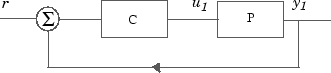## System with Uncertain Parameters

As an example of a closed-loop system with uncertain parameters, consider the two-cart "ACC Benchmark" system  consisting of two frictionless carts connected by a spring shown as follows.

ACC Benchmark ProblemThe system has the block diagram model shown below, where the individual carts have the respective transfer functions.

`$\begin{array}{l}{G}_{1}\left(s\right)=\frac{1}{{m}_{1}{s}^{2}}\\ {G}_{2}\left(s\right)=\frac{1}{{m}_{2}{s}^{2}}.\end{array}$`

The parameters m1, m2, and k are uncertain, equal to one plus or minus 20%:

```m1 = 1 ± 0.2 m2 = 1 ± 0.2 k = 1 ± 0.2 ```

"ACC Benchmark" Two-Cart System Block Diagram y1 = P(s) u1The upper dashed-line block has transfer function matrix F(s):

`$F\left(s\right)=\left[\begin{array}{c}0\\ {G}_{1}\left(s\right)\end{array}\right]\left[\begin{array}{cc}1& -1\end{array}\right]+\left[\begin{array}{c}1\\ -1\end{array}\right]\left[\begin{array}{cc}0& {G}_{2}\left(s\right)\end{array}\right].$`

### Build the Uncertain Model

To build the uncertain model of the ACC Benchmark two-cart system, first create uncertain real parameters for the masses and spring constant.

```m1 = ureal('m1',1,'percent',20); m2 = ureal('m2',1,'percent',20); k = ureal('k',1,'percent',20);```

Next, create the transfer functions for each cart.

```s = zpk('s'); G1 = ss(1/s^2)/m1; G2 = ss(1/s^2)/m2;```

Use model arithmetic to assemble F(s), and connect F(s) to the spring k.

```F = [0;G1]*[1 -1]+[1;-1]*[0,G2]; P = lft(F,k)```
```Uncertain continuous-time state-space model with 1 outputs, 1 inputs, 4 states. The model uncertainty consists of the following blocks: k: Uncertain real, nominal = 1, variability = [-20,20]%, 1 occurrences m1: Uncertain real, nominal = 1, variability = [-20,20]%, 1 occurrences m2: Uncertain real, nominal = 1, variability = [-20,20]%, 1 occurrences Type "P.NominalValue" to see the nominal value and "P.Uncertainty" to interact with the uncertain elements. ```

The resulting `P` is a SISO uncertain state-space (`uss`) object with four states and three uncertain parameters, `m1`, `m2`, and `k`. You can obtain the nominal plant from the `NominalValue` property.

`Pnom = zpk(P.NominalValue)`
```Pnom = 1 ------------- s^2 (s^2 + 2) Continuous-time zero/pole/gain model. ```

Suppose that the uncertain model P(s) has a negative feedback controller with the following transfer function.

`$\mathit{C}\left(\mathit{s}\right)=\frac{{100\left(\mathit{s}+1\right)}^{3}}{{\left(0.001\mathit{s}+1\right)}^{3}}$`

Form the closed-loop transfer function from r to y1 with P(s) and P(s) in the standard configuration.```C = 100*ss((s+1)/(.001*s+1))^3; T = feedback(P*C,1); ```

View the closed-loop step response on the time interval from t = 0 s to t = 0.1 s. Use `usample` to generate Monte Carlo random samples of ten combinations of the three uncertain parameters and plot the response. The result gives a sense of the range of responses and the robustness of the system against variations in the uncertain values.

`stepplot(usample(T,10),.1);`